## Lambert's W-Function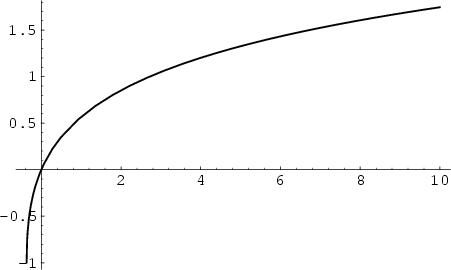The inverse of the function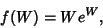(1)

also called the Omega Function. The function is implemented as the Mathematica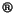(Wolfram Research, Champaign, IL) function ProductLog[z].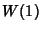is called the Omega Constant and can be considered a sort of Golden Ratio'' of exponentials since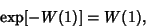(2)

giving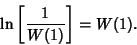(3)

Lambert's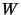-Function has the series expansion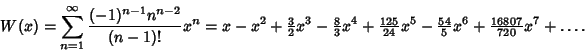(4)

The Lagrange Inversion Theorem gives the equivalent series expansion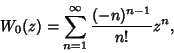(5)

where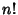is a Factorial. However, this series oscillates between ever larger Positive and Negative values for Real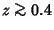, and so cannot be used for practical numerical computation. An asymptotic Formula which yields reasonably accurate results foris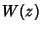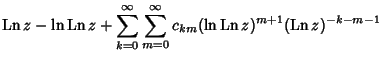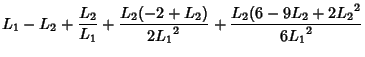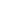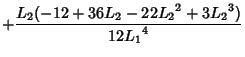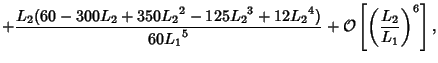(6)

where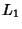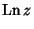(7)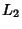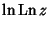(8)

(Corless et al.), correcting a typographical error in de Bruijn (1961). Another expansion due to Gosper is the Double Sum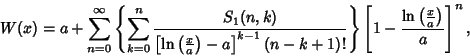(9)

where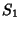is a nonnegative Stirling Number of the First Kind andis a first approximation which can be used to select between branches. Lambert's-function is two-valued for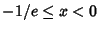. For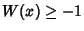, the function is denoted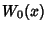or simply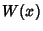, and this is called the principal branch. For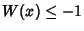, the function is denoted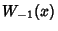. The Derivative ofis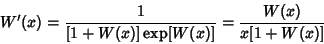(10)

for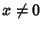. For the principal branch when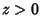,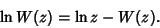(11)